## Bessel Differential Equation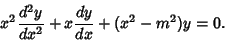(1)

Equivalently, dividing through by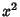,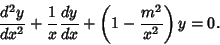(2)

The solutions to this equation define the Bessel Functions. The equation has a regular Singularity at 0 and an irregular Singularity at.

A transformed version of the Bessel differential equation given by Bowman (1958) is(3)

The solution is(4)

where(5)and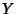are the Bessel Functions of the First and Second Kinds, and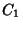andare constants. Another form is given by letting,, and(Bowman 1958, p. 117), then(6)

The solution is(7)

See also Airy Functions, Anger Function, Bei, Ber, Bessel Function, Bourget's Hypothesis, Catalan Integrals, Cylindrical Function, Dini Expansion, Hankel Function, Hankel's Integral, Hemispherical Function, Kapteyn Series, Lipschitz's Integral, Lommel Differential Equation, Lommel Function, Lommel's Integrals, Neumann Series (Bessel Function), Parseval's Integral, Poisson Integral, Ramanujan's Integral, Riccati Differential Equation, Sonine's Integral, Struve Function, Weber Functions, Weber's Discontinuous Integrals

References

Bowman, F. Introduction to Bessel Functions. New York: Dover, 1958.

Morse, P. M. and Feshbach, H. Methods of Theoretical Physics, Part I. New York: McGraw-Hill, p. 550, 1953.